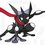# Shapes, the confusion finally solved! (notes) P.S. This note only talks about trapezoids and circles.

Circles: Why are circles so mysterious? How do you calculate their pie, area, radius, or circumference? Well, in this note, I will explain in a painstakingly long note typed by me (hopefully not). Ready? Grab your notebook or bookmark this page, and if you know how to calculate all this already, you can skip right on to the problem I will post shortly after!

Word dictionary: Circumference(c): The perimeter of the circle (can be calculated using the equation c = pi x d) Chord: A line segment whose endpoints are on the circle Diameter(d): Basically a chord running right through the middle of the circle Radius(r): Cut the diameter of a circle in half, and BOOM, you get the radius of the circle Pi: The ratio of a circle's circumference to its diameter (pi = circumference/diameter or pi = c/d) We can't know the exact pi in most circles, we can use the power ofLol anyway lets get started!

Problem #1 (d/r): A circle has a diameter of 1.5. What is it's radius? A radius is half the size of a diameter, or r = d/2, or in this case, r = 1.5/2, so r = .75.

Problem #2 (Pi/c/d): Just for this problem, lets pretend that the approximation of pi is 6.11. we still haven't found the circumference of the imaginary circle, so lets do that. Using the equation in the word dictionary and the diameter from above (in problem 1 aka d = 1.5), can you find the circumference of the circle? Answer: 6.11 x 1.5 = 9.165.

Problem #3 (Pi/c/d): Okay, you might think this is a repeat of problem 2, but trust me, it's not. Lets move on to a new circle with a diameter of 6 and a circumference of 4. What is the value of Pi? Answer: 4/6 = 2/3 = %66.666666666666....

Trapezoids: Phew, we're already done with circles. Fortunately for me, there isn't much to talk about when it comes to trapezoids :). Anyway, lets dive in!

Word dictionary: Base 1 (b1): The top of the trapezoid Base 2 (b2): The bottom of the trapezoid Height (h): The height of the trapezoid Area (a): Can be calculated by the equation (a = b1+b2/2 x h)

WARNING: THERE IS ONLY ONE PROBLEM IN THE TRAPEZOID SECTION) Problem #1 (b1/b2/h): A trapezoid has a top length of 4 ft and a bottom length of 8 ft. It's height is 3 ft. What is it's area? Answer: a = 4+8/2 x 3 = 18 ft.Note by Ash Ketchup
4 months, 2 weeks ago

This discussion board is a place to discuss our Daily Challenges and the math and science related to those challenges. Explanations are more than just a solution — they should explain the steps and thinking strategies that you used to obtain the solution. Comments should further the discussion of math and science.

When posting on Brilliant:

• Use the emojis to react to an explanation, whether you're congratulating a job well done , or just really confused .
• Ask specific questions about the challenge or the steps in somebody's explanation. Well-posed questions can add a lot to the discussion, but posting "I don't understand!" doesn't help anyone.
• Try to contribute something new to the discussion, whether it is an extension, generalization or other idea related to the challenge.

MarkdownAppears as
*italics* or _italics_ italics
**bold** or __bold__ bold
- bulleted- list
• bulleted
• list
1. numbered2. list
1. numbered
2. list
Note: you must add a full line of space before and after lists for them to show up correctly
paragraph 1paragraph 2

paragraph 1

paragraph 2

[example link](https://brilliant.org)example link
> This is a quote
This is a quote
    # I indented these lines
# 4 spaces, and now they show
# up as a code block.

print "hello world"
# I indented these lines
# 4 spaces, and now they show
# up as a code block.

print "hello world"
MathAppears as
Remember to wrap math in $$ ... $$ or $ ... $ to ensure proper formatting.
2 \times 3 $2 \times 3$
2^{34} $2^{34}$
a_{i-1} $a_{i-1}$
\frac{2}{3} $\frac{2}{3}$
\sqrt{2} $\sqrt{2}$
\sum_{i=1}^3 $\sum_{i=1}^3$
\sin \theta $\sin \theta$
\boxed{123} $\boxed{123}$